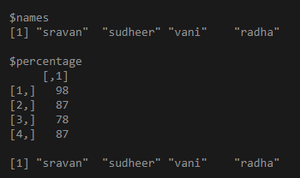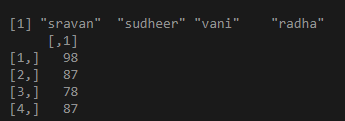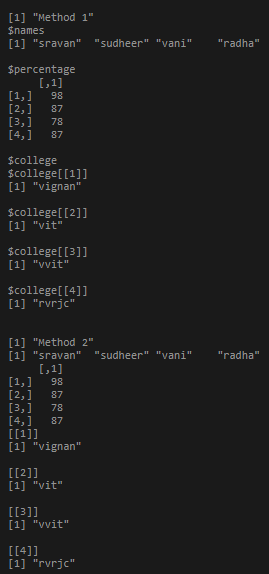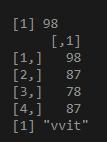GFG App
Open AppBrowser
Continue

In this article, we are going to create a list of elements and access those columns in R. We are first creating a list with matrix and vectors and access those columns using R.

Approach

• Create a list

Syntax:

list_name=list(var1, var2, varn..)

• Assign names to list elements as columns names. We can give names using names() function

Syntax:

names(list_name)=c(var1, var2, varn)

• Access those columns and elements.

### Simple list

Method 1: Using indices

In this method, we simply have to pass the index of the column with the name of the list to extract that specific column.

Example:

## R

 `# Create a list that can hold a vector, and a matrix ` `list1 <- ``list``(``c``(``"sravan"``, ``"sudheer"``, ``"vani"``, ``"radha"``),  ` `              ``matrix``(``c``(98, 87, 78, 87))) ` ` `  `# assign names to the elements in the list. ` `names``(list1) <- ``c``(``"names"``, ``"percentage"``) ` ` `  `# access the column 1 ` `print``(list1) ` ` `  `# access the column 2 ` `print``(list1) `

Output:Method2: Using \$ operator.

In this method, the name of the column to be retrieved has to be passed with its name and name of the list separated by the dollar sign(\$).

Syntax:

list_name\$column_name

Example:

## R

 `# Create a list that can hold a vector, and a matrix ` `list1 <- ``list``(``c``(``"sravan"``, ``"sudheer"``, ``"vani"``, ``"radha"``),  ` `              ``matrix``(``c``(98, 87, 78, 87))) ` ` `  `# assign names to the elements in the list. ` `names``(list1) <- ``c``(``"names"``, ``"percentage"``) ` ` `  `# access the column 1 ` `print``(list1\$names) ` ` `  `# access the column 2 ` `print``(list1\$percentage) `

Output:## A list with different structures

A list can contain a matrix, vector, and a list as arguments to a list but to access them the method remains the same and it has been discussed in the code below.

Example:

## R

 `# Create a list that can hold a vector, and a matrix and a list ` `list1 <- ``list``(``c``(``"sravan"``, ``"sudheer"``, ``"vani"``, ``"radha"``),  ` `              ``matrix``(``c``(98, 87, 78, 87)), ` `              ``list``(``'vignan'``, ``'vit'``, ``'vvit'``, ``'rvrjc'``)) ` ` `  `# assign names to the elements in the list. ` `names``(list1) <- ``c``(``"names"``, ``"percentage"``, ``"college"``) ` ` `  `print``(``"Method 1"``) ` ` `  `# access the column 1 ` `print``(list1) ` ` `  `# access the column 2 ` `print``(list1) ` ` `  `# access the column 3 ` `print``(list1) ` ` `  `print``(``"Method 2"``) ` ` `  `# access the column 1 ` `print``(list1\$names) ` ` `  `# access the column 2 ` `print``(list1\$percentage) ` ` `  `# access the column 3 ` `print``(list1\$college)`

Output:It is possible to access nested elements using [[]] operator.

Syntax:

list_name[[value]][[value]]…

Example:

## R

 `# Create a list that can hold a vector, and a  ` `# matrix and a list ` `list1 <- ``list``(``c``(``"sravan"``, ``"sudheer"``, ``"vani"``, ``"radha"``),  ` `              ``matrix``(``c``(98, 87, 78, 87)), ` `              ``list``(``'vignan'``, ``'vit'``, ``'vvit'``, ``'rvrjc'``)) ` ` `  `# access 2nd column first element ` `print``(list1[][]) ` ` `  `# access 2nd column  ` `print``(list1[]) ` ` `  `# access 3rd column third element ` `print``(list1[][]) `

Output:My Personal Notes arrow_drop_up